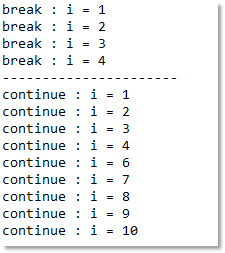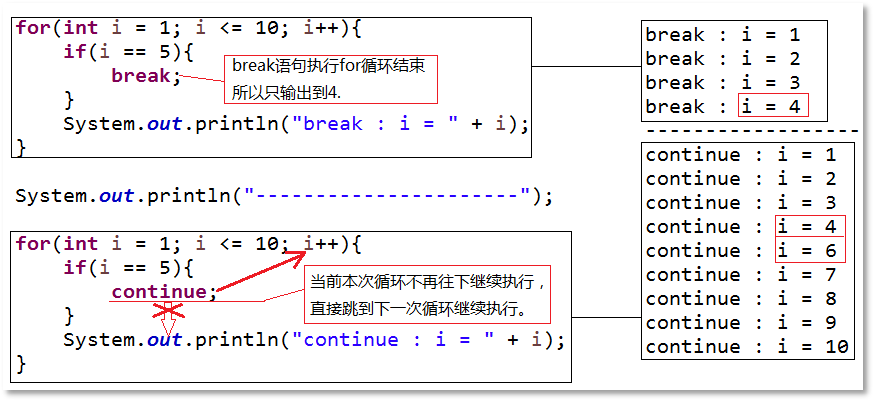java转向语句之break和continue

break语句

java.util.Scanner scanner = new java.util.Scanner(System.in);
int sum = 0;
while(true){
System.out.print("请输入[0-100]的整数：");
int num = scanner.nextInt();
if(num >= 0 && num <= 100){
sum += num;
System.out.println("累加" + num + "成功！");
}else{
System.out.println("不在合法范围，无法累加" + num);
break;
}
}
System.out.println("最终累加结果 = " + sum);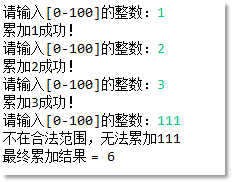for(int j = 1; j <= 2; j++){
for(int i = 1; i <= 10 ; i++){
if(i == 5){
break;
}
System.out.println("i = " + i);
}
}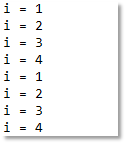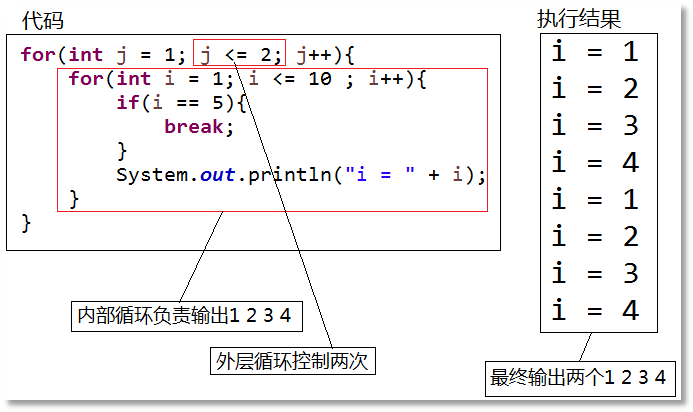first:for(int j = 1; j <= 2; j++){
for(int i = 1; i <= 10 ; i++){
if(i == 5){
break first;
}
System.out.println("i = " + i);
}
}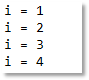continue语句

continue语句也是单个单词自成一条java语句，例如“continue;”，它和break语句都是用来控制循环的，break语句是用来终止循环的执行，而continue语句则是用来终止当前本次循环，直接进入下一次循环继续执行。请参照以下代码以及运行结果进行对比学习：

for(int i = 1; i <= 10; i++){
if(i == 5){
break;
}
System.out.println("break : i = " + i);
}

System.out.println("----------------------");

for(int i = 1; i <= 10; i++){
if(i == 5){
continue;
}
System.out.println("continue : i = " + i);
}